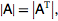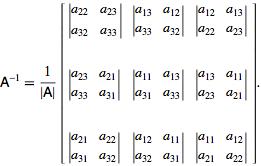# Show matrix has no inverse

t_n_p
[SOLVED] Show matrix has no inverse

## Homework Statement

Show that for a matrix A that has dimensions n by n (where n is an odd number) and is skew symmetric (i.e. transpose of A = -A) that it has no inverse

## The Attempt at a Solution

Not sure where to start but have a feeling I have to used the definition transpose of A = -A to find the determinant first.

Staff Emeritus
Not sure where to start but have a feeling I have to used the definition transpose of A = -A to find the determinant first.
Good idea. If you can relate the determinant of A to the determinant of -A, then you may have a chance.

t_n_p
For starters, I've decided to choose n=3. When creating matrix A and hence -A, should I just nominate values of a,b,c etc and have the determinant in terms of each matrix entry like I've shown below? Where to from here?

http://img74.imageshack.us/img74/9438/59195012ss6.jpg [Broken]

Last edited by a moderator:
Staff Emeritus
For starters, I've decided to choose n=3. When creating matrix A and hence -A, should I just nominate values of a11, a12, a13 etc and have the determinant in terms of each matrix entry?

No. You should keep n as an arbitrary odd number, and keep A an arbitrary matrix, if you want to answer the question.

Hint: How are the determinant of a matrix and its transpose related?

fikus
try to look what is
$$A^T A$$
then use the rule for determinant of product.

fikus
uhh sorry, just look what determinant of transpose matrix is as cristo said.

t_n_p
No. You should keep n as an arbitrary odd number, and keep A an arbitrary matrix, if you want to answer the question.

Hint: How are the determinant of a matrix and its transpose related?What then?Last edited by a moderator:
Staff Emeritus
Gold MemberWhat then?Correct. You have yet to make use if this information,
(i.e. transpose of A = -A)

Last edited by a moderator:
t_n_p
Ok, so
|A| = |transpose of A| = |-A|

But how do I show no inverse exists?

Gold Member
|-A|=(-1)^n|A|
but you need to know this before using this.
and there is no other way here.

Staff Emeritus
|-A|=(-1)^n|A|
but you need to know this before using this.
and there is no other way here.

You should not give solutions to homework problems (and this is, in essence, a solution).

You can give hints, or suggestions of ways to attack the problem. For example, one could ask if the OP could express det(pA) in terms of det(A), in the case of an nxn matrix. The result then follows on setting p=-1. However, just giving a formula does not help the student learn anything.

t_n_p
so now
|A| = |transpose of A| = |-A| = (-1)^n|A|

I still don't get it

Staff Emeritus
Gold Member
so now
|A| = |transpose of A| = |-A| = (-1)^n|A|

Still can't see how to piece it all together though..
t_n_p, as cristo has said, you have virtually been given the answer by LQG. Try thinking about what has been said for a while before asking further questions. We won't hold you're hand through every single step of every single question, you have to do some work.

t_n_p
I've thought about it. The formula provided by LQG does not register anything to me. Yes, I knowand the determinant of A can supposedly be found using the formula provided by LQG, but my denominator thus appears merely as (-1)^n|A|. I am also unfamiliar with raising constants to the power of a matrix. I can not see how this is relevant or useful to proving the inverse does not exist. Hence my question is how is it related to what I want to know?

#### Attachments

Staff Emeritus
What is the condition for the existence of the inverse to a matrix, in terms of the determinant? This is something you learn in the first few weeks of a linear algebra course, so if you're not sure, then look through your course notes, or textbook.

Gold Member
You should not give solutions to homework problems (and this is, in essence, a solution).

You can give hints, or suggestions of ways to attack the problem. For example, one could ask if the OP could express det(pA) in terms of det(A), in the case of an nxn matrix. The result then follows on setting p=-1. However, just giving a formula does not help the student learn anything.

yes, but from his posts here you can see that he doesn't recall this equation, this is why i urged him to prove it and then use it, if he just uses then he will not learn anything.

t_n_p
for the inverse to exist, determinant must not equal zero. So if I set the equation given by LQG and equate to zero, the inverse will not exist?

i.e. (-1)^n|A| = 0

Last edited:
Staff Emeritus
Gold Member
for the inverse to exist, determinant must not equal zero.
Correct. You have a further condition on the matrix which you have not yet used.

Staff Emeritus
So if I set the equation given by LQG and equate to zero, the inverse will not exist?

i.e. (-1)^n|A| = 0

No, now's the time you need to think a little. Why are you setting that to zero? It seems like you're just doing it to satisfy the condition!

You know that, since the matrix is skew-symmetric, that det(A)=det(-A). You also have the expression that lqg gave you: det(-A)=(-1)^n.det(A). Put these two together, and what do you get?

I'm not sure how I can help any more without explicitly telling you the answer!

t_n_p
Ok, I think I'm looking too hard into this.

Basically I want to show that |A| = 0, and hence the inverse does not exist.

I know that
http://img142.imageshack.us/img142/876/83639370us7.jpg [Broken]

Say I ignore |-A| for the time being, and therefore I have |A| = (-1)^n|A|. I then divide both sides by |A| and take log10 both sides. Am I on the right track?

Last edited by a moderator:
Staff Emeritus
What you have is det(A)=det(-A)=(-1)^n.det(A) (note that det(A) is not in the exponent!)

What do you know about n? What does the equation reduce to on utilising this condition?

t_n_p
pffft no wonder.

|A| = |-A| = (-1)^n . |A|,

since n is odd,
|A| = |-A| = -|A|

|A| = |-A| + |A|

|-A| = 0

since |-A| = |A|,

|A| = 0 and hence inverse does not exist

Last edited:
Staff Emeritus
pffft no wonder.

|A| = (-1)^n . |A|
1 = (-1)^n

You know you can only cancel something from both sides if that thing is not zero. Here you want to show it is zero, so you can't cancel it!

Anyway, you have det(A)=-det(A). Under what conditions does this apply?

Last edited:
t_n_p
I've edited my above post, is your post still relevant?

Let's start from scratch.

|A| = |transpose A|

since A is skew symmetric we also know
|transpose A| = |-A|

therefore, |A| = |transpose A| = |-A|

also |-A| = (-1)^n. |A|

therefore |A| = |transpose A| = |-A|= (-1)^n. |A|

since n is odd,
|A| = |transpose A| = |-A|= (-1)|A|

Last edited:
Staff Emeritus
I've edited my above post, is your post still relevant?

t_n_p said:
Let's start from scratch.

|A| = |transpose A|

since A is skew symmetric we also know
|transpose A| = |-A|

therefore, |A| = |transpose A| = |-A|

also |-A| = (-1)^n. |A|

therefore |A| = |transpose A| = |-A|= (-1)^n. |A|

since n is odd,
|A| = |transpose A| = |-A|= (-1)|A|
Ok, so now you're up to my last post:
me said:
Anyway, you have det(A)=-det(A). Under what conditions does this apply?

t_n_p
I've looked through my notes/textbook, can't seem to locate when |A| = -|A|. Google search says something about left handed matrix?

Staff Emeritus
I've looked through my notes/textbook, can't seem to locate when |A| = -|A|. Google search says something about left handed matrix?

This is just algebra, there's nothing special to it. The determinant of a matrix is an integer, let's say d. So, you want to know for which integer does d=-d or for which integer does 2d=0?

t_n_p
when d = 0, therefore |A| = -|A| ONLY when |A| = 0

since |A| = 0, inv(A) does not exist.

SOLVED.
Thanks Cristo, appreciate it mate.

Staff Emeritus